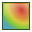# FE Card

This card controls the calculation of near fields.

On the Request tab, in the Solution requests group, click theNear field (FE) icon.

## Parameters:

Request name
The name of the request.
Select what to calculate
No field calculation
Field is not calculated.
Electric field values
Calculate the electric field, $E$ .
Magnetic field values
Calculate the magnetic field, $H$ .
Both electric field and magnetic field values
Calculate both electric and magnetic fields.
Electric field and SAR values (in cuboids)
Calculate the electric field and SAR values in the dielectric volume elements. For this option, no other parameters are required.
Electric vector potential
Calculate the electric vector potential, $F$ .
Electric scalar potential
Calculate the electric scalar potential, $\phi$ .
Gradient of the scalar electric potential
Calculate the gradient of the scalar electric potential, $\nabla \phi$ .
Magnetic vector potential
Calculate the magnetic vector potential, $A$ .
Magnetic scalar potential
Calculate the magnetic scalar potential, $\psi$ .
Gradient of the scalar magnetic potential
Calculate the gradient of the scalar magnetic potential, $\nabla \psi$ .
Use old output format
If this item is checked, the old format of the near field is used in the output file. This should only be used for compatibility with third party post processors. (POSTFEKO cannot extract SAR values from near fields in this format).
Calculate only the scattered part of the field
When this item is checked only the scattered part of the field/potential is computed and written to the output file. Otherwise the total field/potential, that is the sum of the scattered and source contributions will be computed.
Note: Depending on the formulation used in Feko the region where an impressed source is regarded as the incident field could differ.
For example when using the surface equivalence principle in the MoM to model dielectric bodies each source will act as the incident field only in that medium where the source is located. To elaborate, consider an SEP solution of a Hertzian dipole inside a dielectric body, A. In a nearby dielectric body, B (different label but could have the same or different medium properties), this source would not be considered an impressed source.
Coordinate system
In this group, the coordinate system for the calculation of the requested fields is specified.
By selecting Cartesian, Cartesian boundary, Cylindrical, Spherical, Cylindrical (X axis), Cylindrical (Y axis) and Conical, additional groups will be shown for Starting values, Increment and No. of points.
If Specified points is selected, the No. of field points and the Coordinates of each near field point are required.
If Tetrahedral mesh is selected, the near field is calculated at the vertices and edge mid-points of the tetrahedra. No additional information is required.
Note: All coordinates are in metres and all angles in degrees.

Scaling with the SF card is only applicable when the option Modify all dimension related values is checked in the SF card (default behaviour and highly recommended). In this case coordinates must be in metres after scaling.

Potentials cannot be computed with the FE card in the following cases:
• The UTD solver is used.
• The PO solver is used.
• The Green's functions for layered spheres or multi-layered planar media is used (but the free space Green's function is supported).

If the total potentials are requested, the potentials for the sources are added. These are not available for a plane wave (A0 card) or an impressed radiation pattern (AR card) and Feko will give an error in this regard. For a magnetic dipole (A6 card) the electric ring current model yields $A$ and the magnetic current yields $F$ and $\nabla \psi$ . All the other potentials mentioned in First drop-down list are zero.

If output to .efe and/or .hfe files is requested with the DA card, then $A$ and $\nabla \phi$ are written to the .efe file, while $F$ and $\nabla \psi$ are written to the .hfe file.

If a ground plane is used, calculation of the near fields in/below the ground plane is not possible. Requested points in the area z < 0 will be ignored.

The coordinates may be offset with the OF card. The OF card allows the near field on the surface of a sphere to be calculated where the centre of the sphere is not located at the origin of the coordinate system.